If you want to land a job in data science, you’ll need to pass a rigorous and competitive interview process. In fact, most top companies will have at least 3 rounds of interviews.

During the process, you’ll be tested for a variety of skills, including:

• Your technical and programming skills
• Your ability to structure solutions to open-ended problems
• Your ability to apply machine learning effectively
• Your ability to analyze data with a range of methods
• Your communication skills, cultural fit, etc.
• And your mastery of key concepts in data science and machine learning (← this is the focus of this post)

In this post, we’ll provide some examples of machine learning interview questions and answers. But before we get to them, there are 2 important notes:

1. This is not meant to be an exhaustive list, but rather a preview of what you might expect.
2. The answers are meant to be concise reminders for you. If it’s the first time you’ve seen a concept, you’ll need to research it more in order for the answer to make sense.

The following questions are broken in 9 major topics.## 1. The Big Picture

Essential ML theory, such as the Bias-Variance tradeoff.

#### 1.1 - What are parametric models? Give an example.

Parametric models are those with a finite number of parameters. To predict new data, you only need to know the parameters of the model. Examples include linear regression, logistic regression, and linear SVMs.

Non-parametric models are those with an unbounded number of parameters, allowing for more flexibility. To predict new data, you need to know the parameters of the model and the state of the data that has been observed. Examples include decision trees, k-nearest neighbors, and topic models using latent dirichlet analysis.

#### 1.2 - What is the "Curse of Dimensionality?"

The difficulty of searching through a solution space becomes much harder as you have more features (dimensions).

Consider the analogy of looking for a penny in a line vs. a field vs. a building. The more dimensions you have, the higher volume of data you'll need.

#### 1.3 - Explain the Bias-Variance Tradeoff.

Predictive models have a tradeoff between bias (how well the model fits the data) and variance (how much the model changes based on changes in the inputs).

Simpler models are stable (low variance) but they don't get close to the truth (high bias).

More complex models are more prone to being overfit (high variance) but they are expressive enough to get close to the truth (low bias).

The best model for a given problem usually lies somewhere in the middle.## 2. Optimization

Algorithms for finding the best parameters for a model.

#### 2.1 - What is the difference between stochastic gradient descent (SGD) and gradient descent (GD)?

Both algorithms are methods for finding a set of parameters that minimize a loss function by evaluating parameters against data and then making adjustments.

In standard gradient descent, you'll evaluate all training samples for each set of parameters. This is akin to taking big, slow steps toward the solution.

In stochastic gradient descent, you'll evaluate only 1 training sample for the set of parameters before updating them. This is akin to taking small, quick steps toward the solution.

#### 2.2 - When would you use GD over SDG, and vice-versa?

GD theoretically minimizes the error function better than SGD. However, SGD converges much faster once the dataset becomes large.

That means GD is preferable for small datasets while SGD is preferable for larger ones.

In practice, however, SGD is used for most applications because it minimizes the error function well enough while being much faster and more memory efficient for large datasets.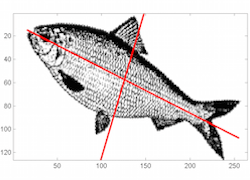## 3. Data Preprocessing

Dealing with missing data, skewed distributions, outliers, etc.

#### 3.1 - What is the Box-Cox transformation used for?

The Box-Cox transformation is a generalized "power transformation" that transforms data to make the distribution more normal.

For example, when its lambda parameter is 0, it's equivalent to the log-transformation.

It's used to stabilize the variance (eliminate heteroskedasticity) and normalize the distribution.

#### 3.2 - What are 3 data preprocessing techniques to handle outliers?

1. Winsorize (cap at threshold).
2. Transform to reduce skew (using Box-Cox or similar).
3. Remove outliers if you're certain they are anomalies or measurement errors.

#### 3.3 - What are 3 ways of reducing dimensionality?

1. Removing collinear features.
2. Performing PCA, ICA, or other forms of algorithmic dimensionality reduction.
3. Combining features with feature engineering.## 4. Sampling & Splitting

How to split your datasets to tune parameters and avoid overfitting.

#### 4.1 - How much data should you allocate for your training, validation, and test sets?

You have to find a balance, and there's no right answer for every problem.

If your test set is too small, you'll have an unreliable estimation of model performance (performance statistic will have high variance). If your training set is too small, your actual model parameters will have high variance.

A good rule of thumb is to use an 80/20 train/test split. Then, your train set can be further split into train/validation or into partitions for cross-validation.

#### 4.2 - If you split your data into train/test splits, is it still possible to overfit your model?

Yes, it's definitely possible. One common beginner mistake is re-tuning a model or training new models with different parameters after seeing its performance on the test set.

In this case, its the model selection process that causes the overfitting. The test set should not be tainted until you're ready to make your final selection.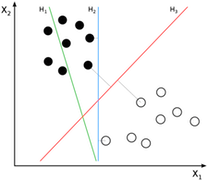## 5. Supervised Learning

Learning from labeled data using classification and regression models.

#### 5.1 - What are the advantages and disadvantages of decision trees?

Advantages: Decision trees are easy to interpret, nonparametric (which means they are robust to outliers), and there are relatively few parameters to tune.

Disadvantages: Decision trees are prone to be overfit. However, this can be addressed by ensemble methods like random forests or boosted trees.

#### 5.2 - What are the advantages and disadvantages of neural networks?

Advantages: Neural networks (specifically deep NNs) have led to performance breakthroughs for unstructured datasets such as images, audio, and video. Their incredible flexibility allows them to learn patterns that no other ML algorithm can learn.

Disadvantages: However, they require a large amount of training data to converge. It's also difficult to pick the right architecture, and the internal "hidden" layers are incomprehensible.

#### 5.3 - How can you choose a classifier based on training set size?

If training set is small, high bias / low variance models (e.g. Naive Bayes) tend to perform better because they are less likely to be overfit.

If training set is large, low bias / high variance models (e.g. Logistic Regression) tend to perform better because they can reflect more complex relationships.## 6. Unsupervised Learning

Learning from unlabeled data using factor and cluster analysis models.

#### 6.1 - Explain Latent Dirichlet Allocation (LDA).

Latent Dirichlet Allocation (LDA) is a common method of topic modeling, or classifying documents by subject matter.

LDA is a generative model that represents documents as a mixture of topics that each have their own probability distribution of possible words.

The "Dirichlet" distribution is simply a distribution of distributions. In LDA, documents are distributions of topics that are distributions of words.

#### 6.2 - Explain Principle Component Analysis (PCA).

PCA is a method for transforming features in a dataset by combining them into uncorrelated linear combinations.

These new features, or principal components, sequentially maximize the variance represented (i.e. the first principal component has the most variance, the second principal component has the second most, and so on).

As a result, PCA is useful for dimensionality reduction because you can set an arbitrary variance cutoff.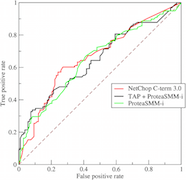## 7. Model Evaluation

Making decisions based on various performance metrics.

#### 7.1 - What is the ROC Curve and what is AUC (a.k.a. AUROC)?

The ROC (receiver operating characteristic) the performance plot for binary classifiers of True Positive Rate (y-axis) vs. False Positive Rate (x-
axis).

AUC is area under the ROC curve, and it's a common performance metric for evaluating binary classification models.

It's equivalent to the expected probability that a uniformly drawn random positive is ranked before a uniformly drawn random negative.

#### 7.2 - Why is Area Under ROC Curve (AUROC) better than raw accuracy as an out-of- sample evaluation metric?

AUROC is robust to class imbalance, unlike raw accuracy.

For example, if you want to detect a type of cancer that's prevalent in only 1% of the population, you can build a model that achieves 99% accuracy by simply classifying everyone has cancer-free.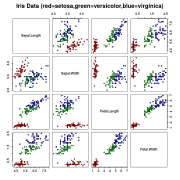## 8. Ensemble Learning

Combining multiple models for better performance.

#### 8.1 - Why are ensemble methods superior to individual models?

They average out biases, reduce variance, and are less likely to overfit.

There's a common line in machine learning which is: "ensemble and get 2%."

This implies that you can build your models as usual and typically expect a small performance boost from ensembling.

#### 8.2 - Explain bagging.

Bagging, or Bootstrap Aggregating, is an ensemble method in which the dataset is first divided into multiple subsets through resampling.

Then, each subset is used to train a model, and the final predictions are made through voting or averaging the component models.

Bagging is performed in parallel.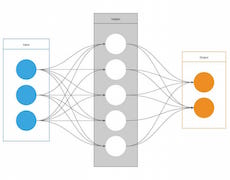How machine learning can help different types of businesses.

#### 9.1 - What are some key business metrics for (S-a-a-S startup | Retail bank | e-Commerce site)?

Thinking about key business metrics, often shortened as KPI's (Key Performance Indicators), is an essential part of a data scientist's job. Here are a few examples, but you should practice brainstorming your own.

• S-a-a-S startup: Customer lifetime value, new accounts, account lifetime, churn rate, usage rate, social share rate
• Retail bank: Offline leads, online leads, new accounts (segmented by account type), risk factors, product affinities
• e-Commerce: Product sales, average cart value, cart abandonment rate, email leads, conversion rate

#### 9.2 - How can you help our marketing team be more efficient?

The answer will depend on the type of company. Here are some examples.

• Clustering algorithms to build custom customer segments for each type of marketing campaign.
• Natural language processing for headlines to predict performance before running ad spend.
• Predict conversion probability based on a user's website behavior in order to create better re-targeting campaigns.

How did you do? Were there any concepts that were unfamiliar to you? If you found any gaps in your knowledge, be sure to spend some extra time preparing!

Remember...

"Fortune favors the prepared mind." ~ Dr. Louis Pasteur